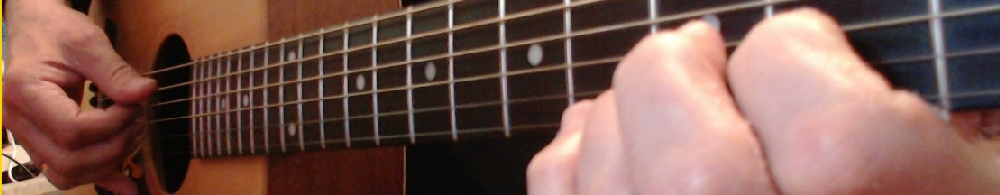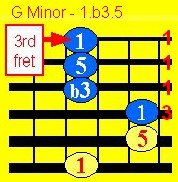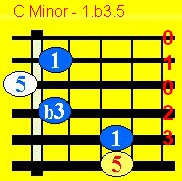﻿ Theory page7Music Slide RuleBasic Practical Theory 77.  UNDERSTANDING FORMULAS CONTINUED..

CHORDS HAVE FORMULAS ALSO

Now for Minor Chords

All Minor Chords have the formula - 1 - b3 - 5

This means that any Minor Chord is made up of the

1st  - b3rd  and 5th notes of it’s Major Scale

Example 1.  Let us look at a C Minor Chord

I     II   III   IV   V  VI VII   I

The C Major Scale is   C - D - E - F - G - A - B - C

The C Minor Chord is  C         Eb      G  So flatten the 3rd note E becomes Eb

So you see the notes and formula of the

C Minor Chord are

Formula   1     b3     5

Notes       C    Eb   GI ALWAYS RELATE ALL FORMULAS TO THE MAJOR SCALENOTE

Example 2.  Let us look at a G Minor Chord

I     II   III   IV   V   VI  VII   I

The G Minor Scale is   G - A - B - C - D - E - F# - G

The G Minor Chord is  G         Bb       D

So you see the notes and formula of the

G Minor Chord are

Formula   1     b3     5

Notes       G    Bb    D

All Minor Chords have the Formula   1     b3     5NOTE: All Major Scales are written using Roman Numerals

I     II   III   IV   V   VI  VII   I

and Chords written with ordinary Numbers such as

1   2   3   4   5   6   7 etc..

This helps to differentiate between

Scale Formulas and Chord FormulasThe C Minor Chord shown on Guitar uses combinations of the  1st - b3rd - and 5th   notes of the

C Major ScaleThe G Minor Chord shown on Guitar uses combinations of the  1st - b3rd - and 5th   notes of the

G Major ScaleBACK
NEXTMusicsliderule.com